﻿ Computer Process System Engineering: Courses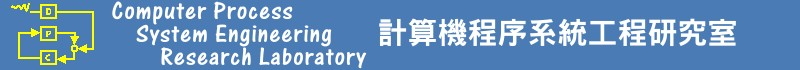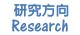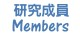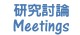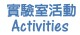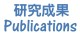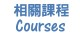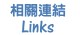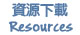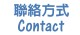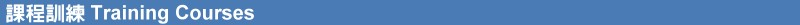• (機械學習於工業的應用) 機械學習與數據分析於化工的應用 (Machine Learning and Data Analysis in Chemical Engineering Applications)

• More and more intelligent services are being introduced for industrial applications, bringing in a new era of information society. Machine Learning (or Deep Learning) is the technology leading this wave of intelligence. This course is designed to provide a thorough grounding in the fundamental methodologies, technologies, mathematics and algorithms of machine learning. The topics of the course draw from classical statistics, from machine learning, from data mining, from Bayesian statistics, and from statistical algorithmic. To avoid giving too many theoretical concepts behind machine learning, a step-by-step approach can help students understanding the basic concepts. we emphasize more on practical code examples and work through example applications.

• The course requires basic knowledge in principles of chemical engineering, numerical methods, probability, statistics, calculus, optimization and some computer programming experience,  although it certainly is advantageous to have prior exposure--not strictly required.
• (Spring 2022) Class Meeting Times & Locations: FRI (10:10~12:40, 工419)
• Office hours: MON (12:00~13:00) and WED (11:00~12:00)
• Course Structure:
• (2-25-2022)
• Introduction to Machine Learning and Data Analytics in Chemical Engineering Application
• Python 3 Installation & Basic Guide
• Learning Python (Anaconda Python)
• (3-4-2022)
• Python Mini Course
• HW1: Steady State Simulation of CSTR
• Giving Computer the Ability to Learn from Data
• Three types of machine learning
• (3-11-2022)
• Giving Computer the Ability to Learn from Data
• Basic terminology and notations
• Roadmap for building machine learning system
• Training Simple Machine Learning Algorithm for Classification
• Artificial neurons
• Perceptron & its objective (itis.data)
• (3-18-2022)
• Training Simple Machine Learning Algorithm for Classification
• Adaptive linear neorons
• (3-25-2022)
•  Training Simple Machine Learning Algorithm for Classification
• Perceptron & its objective
• Adaline & minimizing cost functions with gradient descent
• Feature scaling
• Stochastic gradient descent
• Using Scikit-learn library
• HW1: Steady State Simulation of CSTR (Discussions)
• (4-1-2022)
• Logistic regression
• Overfitting via regulation
• Support vector machine: (1) hard, (2) soft, (3) kernel
• Decision tree
• Info gain
• (4-8-2022)
• Decision trees via random forests
• KNN (Lazy learner)
• Dealing with missing data
• Handling categorical data
• HW2: Classification for operating conditions of CSTR
• (4-15-2022)
• Feature scaling
• Selecting meaningful features
• L1 and L2 regularization
• Sequential backward selection (Greedy algorithm)
• Feature selection with random forests
• (4-22-2022)
• Compressing data via dimensionality reduction
• Unsupervised: principal component analysis
• Supervised: linear discriminate analysis
• (4-29-2022)
• Compressing data via dimensionality reduction
• Supervised: linear discriminate analysis
• Kernel principal component analysis
• Pipeline in Scikit-learn
• (5-6-2022)
• Practices for model evaluation
• K-fold cross-validation (LOOCV)
• Learning and validation curves
• Fine-tuning machine learning models
• Grid search
• Nested cross-validation
• Performance evaluation matrices
• Confusion matrix
• Ensemble learning
• Majority voting
• Bagging (Bootstrap samples)
• HW#2: Simple Classification (Different operating conditions of CSTR)  (Discussions)
• (5-13-2022)
• Regression analysis
• Linear regression
• OLS (Ordinary Least Squares)
• Robust regression (RANSAC)
• Regularized method for regression
• Nonlinear relationships using random forest
• (5-20-2022)
• Multilayer artificial neural network
• Forward propagation
• Backpropagation
• Convergence
• HW#3: Regression Analysis (
• (5-27-2022)
• TensorFlow
• Creating tensor
• Manipulating data type and shape of a tensor
• Math operations to tensors
• Split, Stack, Concatenate tensors
• Creating a TensorFlow dataset
• Shuffle, batch and repeat
• Building an NN model in TensorFlow
• Building an NN model in TensorFlow Keras API
• (6-3--2022)
• Sequential data
• Recurrent neural networks (RNN)
• LSTM, GRU
• TBPTT
• (6-17-2022)
• A deep dive into Tensorflow
• Tensorflow v1 & v2
• Tensorflow variables
• Tensorflow Keras API
• (6-24-2022)
• Oral presentations
• Homework: Python would be used for the programming portions of the assignments. During the first week, a tutorial session would be hosted to jump-start your transition into working in Python
• (3-4-2022) HW#1: Steady State Simulation of CSTR in Python (Due: 3-18-2022)
• (4-8-2022) HW#2: Simple Classification (Different operating conditions of CSTR) (Due: 4-28-2022)
• (5-20-2022)  HW#3: Regression Analysis (Due: 6-09-2022)
• (6-3-2022)  HW#4: Compression & Classification (Due: 6-23-2022)